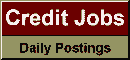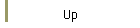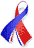DefaultRisk.com the web's biggest credit risk modeling resource.doi> search: A or B Export citation to:- HTML- Text (plain)- BibTeX- RIS- ReDIF

A Formula for Interest Rate Swaps Valuation under Counterparty Risk in presence of Netting Agreements

by Damiano Brigo of Banca IMI,
Massimo Masetti of Banca IMI

May 4, 2005

Abstract: In this document we show how to handle counterparty risk for Interest Rate Swaps (IRS). First we establish a general formula, showing that counterparty risk adds one level of optionality to the contract. Then we introduce the default probabilities using a deterministic intensity model where the default time is modeled as the first jump of a time inhomogeneous Poisson process.

We consider Credit Default Swaps as liquid sources of market default probabilities. We then apply the general formula to a single IRS. The IRS price under counterparty risk turns out to be the sum of swaption prices with different maturities, each weighted with the probability of defaulting around that maturity. Then we consider a portfolio of IRS's in presence of a netting agreement. The related option cannot be valued as a standard swaption, and we resort both to Monte Carlo simulation and to two analytical approximations, investigating them by Monte Carlo simulation under several configurations of market inputs. We find a good agreement between the formula and the simulations in most cases.

The approximated formula is well suited to risk management, where the computational time under each risk factors scenario is crucial and an analytical approximation contains it.

Keywords: Interest Rate Swap, Counterparty Risk Pricing, Netting Agreements, Libor Model.

Books Referenced in this paper:  (what is this?)